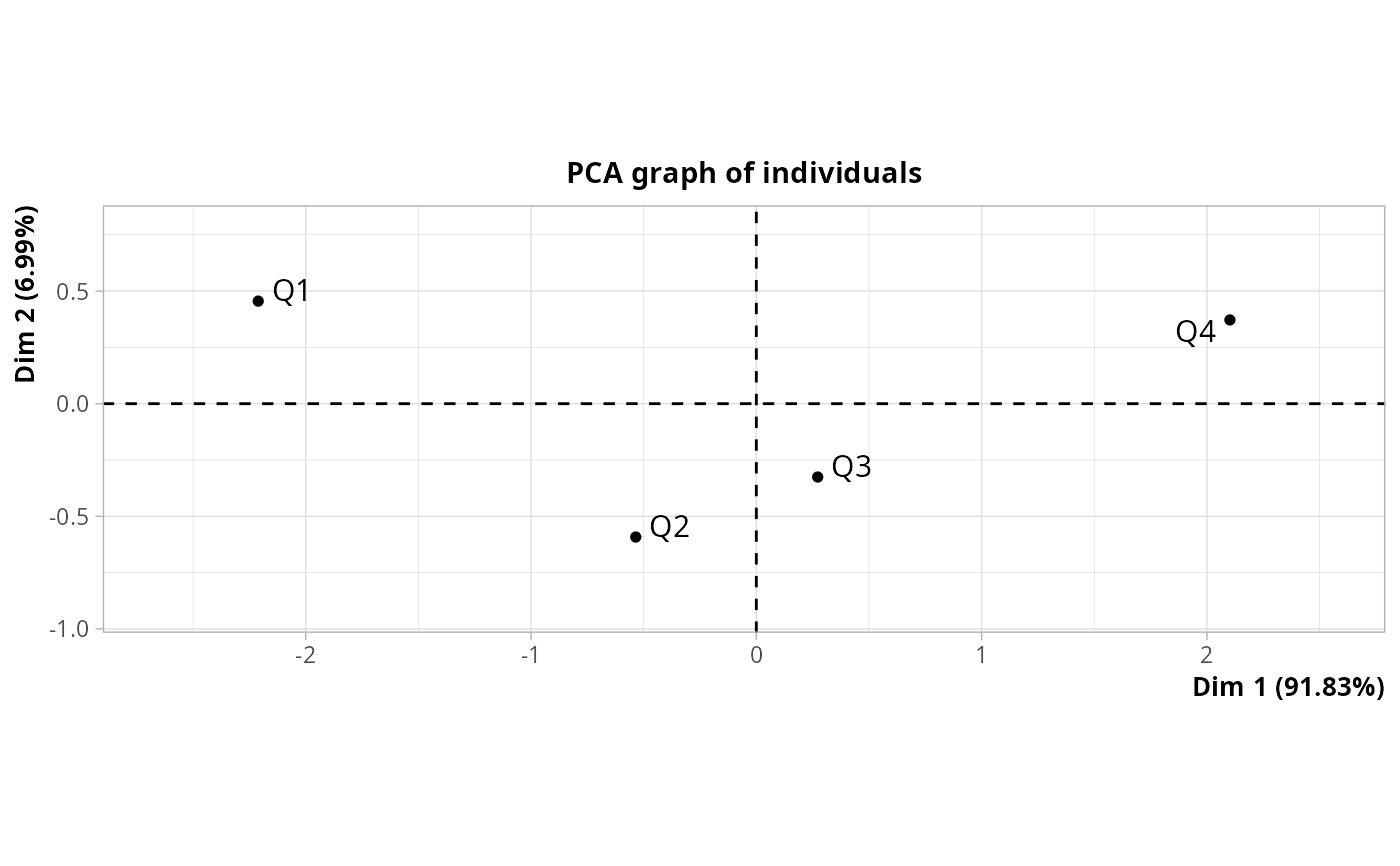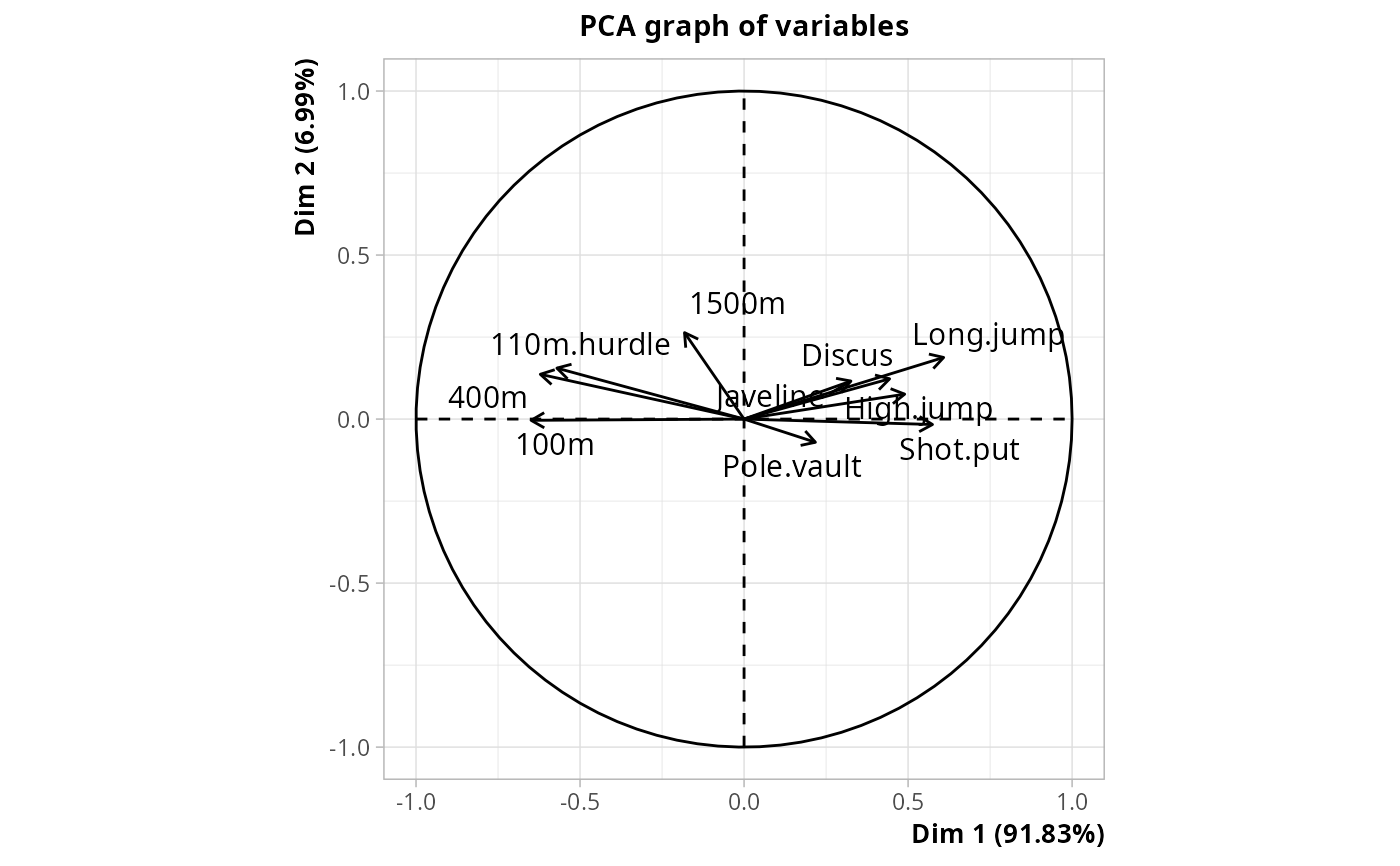Between-class Principal Component Analysis

bcPCA(data, class, row.w = NULL, scale.unit = TRUE, ncp = 5)

## Arguments

data

data frame with only numeric variables

class

factor specifying the class

row.w

numeric vector of row weights. If NULL (default), a vector of 1 for uniform row weights is used.

scale.unit

logical. If TRUE (default) then data are scaled to unit variance.

ncp

number of dimensions kept in the results (by default 5)

## Details

Between-class Principal Component Analysis consists in two steps : 1. Computation of the barycenter of data rows for each category of class 2. Principal Component Analysis of the set of barycenters

It is a quite similar to Linear Discriminant Analysis, but the metric is different.

It can be seen as a special case of PCA with instrumental variables, with only one categorical instrumental variable.

## Value

An object of class PCA from FactoMineR package, with the original data as supplementary individuals, and an additional item :

ratio

the between-class inertia percentage

## Author

Nicolas Robette

PCAiv, DA
library(FactoMineR)
points <- cut(decathlon$Points, c(7300, 7800, 8000, 8120, 8900), c("Q1","Q2","Q3","Q4")) res <- bcPCA(decathlon[,1:10], points) # categories of class plot(res, choix = "ind", invisible = "ind.sup")# variables in decathlon data plot(res, choix = "var")# between-class inertia percentage res$ratio